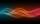# Equatiom

Solve equation with negatives:

X/(-5) + 2 = -9

Result

X =  55

#### Solution:

X/(-5) + 2 = -9

0.2X = 11

X = 55

Calculated by our simple equation calculator.

Leave us a comment of example and its solution (i.e. if it is still somewhat unclear...):

Showing 0 comments:Be the first to comment!#### To solve this example are needed these knowledge from mathematics:

Do you have a linear equation or system of equations and looking for its solution? Or do you have quadratic equation?

## Next similar examples:

1. Find xSolve: if 2(x-1)=14, then x= (solve an equation with one unknown)
2. Simple equation 5Solve equation with fractions: X × 3/8 = 1/2
3. Equation 29Solve next equation: 2 ( 2x + 3 ) = 8 ( 1 - x) -5 ( x -2 )
4. Simple equationSolve the following simple equation: 2. (4x + 3) = 2-5. (1-x)
5. Eq1Solve equation: 4(a-3)=3(2a-5)
6. 15 numberWhat number is smaller (greater) by 15 than its half?
7. Two numbers 6Fill two natural numbers a, b: 7 + blank- blank = 5
8. Mysterious numberThe magician thinks the number: "The mysterious number is first divided by minus five, dividing the result by three, multiplying the number by ten, and dividing the resulting number by minus four. This gives result 5. Can you reveal the mysterious number?
9. Balance of accountTheo had a balance of -\$4 in his savings account. After making a deposit, he has \$25 in his account. What is the overall change to his account?
10. Negative in equation2x + 3 + 7x = – 24, what is the value of x?
11. Forest nurseryIn the forest nursery after winter, they found that 1/10 stems died out of them. For them, they land 193 new spruces. How many spruces are in the forest nursery?
12. Equation 20In given equation: 8/9-4/5=2/9+x, find x
13. NormThree workers planted 3555 seedlings of tomatoes in one dey. First worked at the standard norm, the second planted 120 seedlings more and the third 135 seedlings more than the first worker. How many seedlings were standard norm?
14. Dropped sheetsThree consecutive sheets dropped from the book. The sum of the numbers on the pages of the dropped sheets is 273. What number has the last page of the dropped sheets?
15. Unknown number 11That number increased by three equals three times itself?
16. If-then equationIf 5x - 17 = -x + 7, then x =
17. Whole numbersPavol wrote down a number that is both rational and a whole number. What is one possible number she could have written down?